## Sunday, October 24, 2021

### A CMOS FSK Modem

While researching cassette storage, I found multiple different encodings, the most popular one being Frequency-shift Keying. It simply uses a high tone for a logical one, and a low tone for a zero. We'll be using the standard frequencies of 1,200Hz and 2,400Hz for our two tones.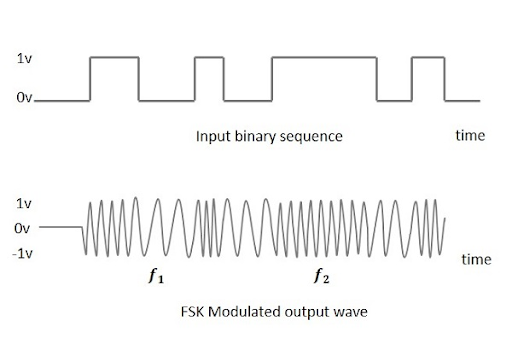FSK encoding - tutorialspoint

Another choice we have to make is regarding phase. If we use two different oscillators for our tones, the phase will be inconsistent between them. This phase difference can cause a sharp "jump" in our output when we switch between them. The jump consists of extra harmonics that can complicate decoding, though they're actually utilised in a related encoding: "Phase-shift Keying".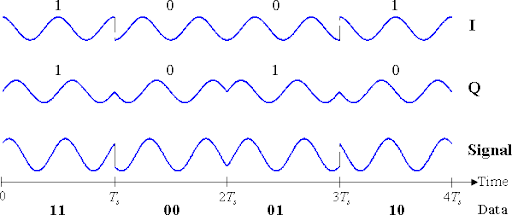Phase discontinuities (from Phase-shift keying) - wikipedia

If we instead change the pitch of a single oscillator, the phase will remain the same, and no extra harmonics will be created. This is called "continuous-phase" and it's the approach we'll be using.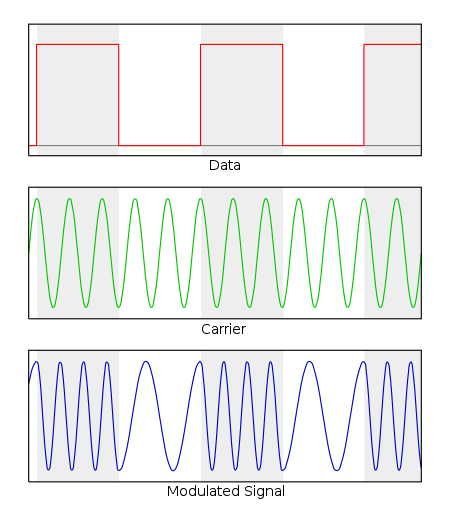The modulated signal is demonstrating continuous-phase - wikipedia

Another factor that can be important is where in the cycle the frequency change occurs. If we switch frequencies at a random point in a cycle, the length of the cycle can be anywhere between that of 1,200Hz and 2,400Hz (833-416uS). Some demodulators aren't affected by this, while some can be. In the interest of easy demodulation, we'll have a consistent switching point at the lowest extreme of the waveform.

## Modulation

So, how do we implement these features into a circuit? We can start with a simple oscillator built around a schmitt trigger. It works by charging a capacitor back and forth between two thresholds. The rate at which it charges/discharges defines the frequency, and that is determined by the resistor/capacitor we select.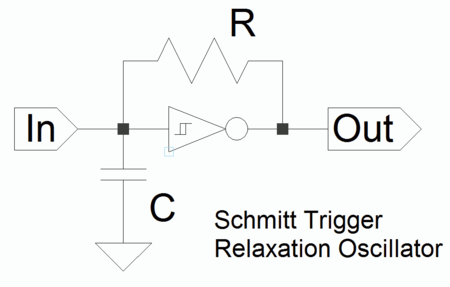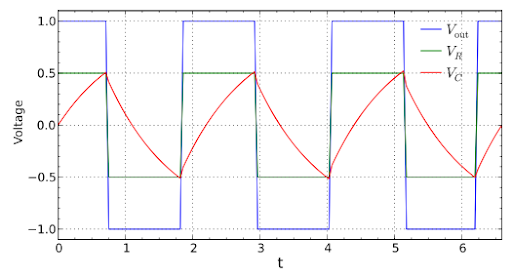capacitor's charge, threshold(s), output - wikipedia

With our particular schmitt trigger, values of 66kΩ and 20nf give us 1,200Hz. If we can halve either of these values, we'll get the other frequency we need, 2,400Hz. There are numerous ways of doing this with analog switches, FETs, etc. Here we will use a bipolar junction transistor to switch a 10nf capacitor in and out. When it's switched "in", it's in parallel with another 10nf capacitor giving an equivalent 20nf (for 1,200Hz). When it's switched "out", we're left with only the 10nf (for 2,400Hz). Now we have digital control over the frequency.

Notice that the frequency is switching in odd spots. In order to limit where in the cycle frequencies can shift, we add a D-type latch. It passes data through only when it sees a rising clock edge. We can use the square output of the schmitt oscillator to provide this clock edge, and the latch's output can control the frequency. This means the frequency can only change at the instant the oscillator begins to rise.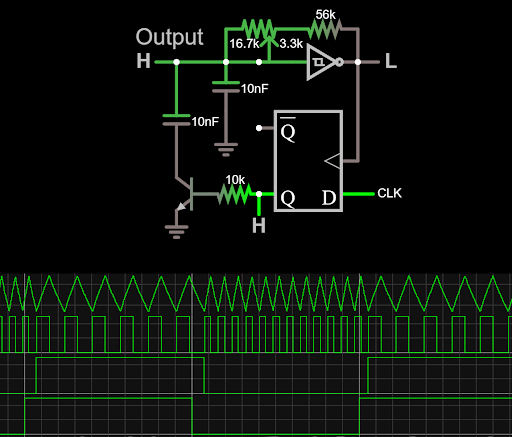link to simulation

Finally, we need to adapt the signal to something a cassette recorder can accept. We use the signal from the oscillator's capacitor, as it's close in amplitude to line level. We buffer it with an op-amp, since we don't want the cassette's input circuitry to load and disturb the capacitor's charging. Following that a capacitor and two resistors form a DC-removing highpass, as well as a voltage divider that gives us an additional mic-level output.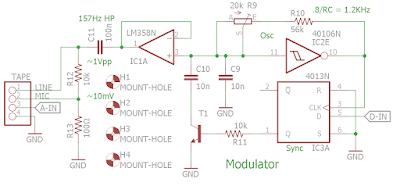our completed modulator schematic

## Demodulator

Write-only memory isn't of much use, so let's work on reading. Some approaches use bandpass filters to determine if the signal contains a 1,200Hz tone or a 2,400Hz tone. We'll instead be detecting the difference in timing between peaks in the signal. This approach can detect frequency in as little as one cycle.

Peaks can be a little tricky to detect, so we're actually going to convert our signal to a square wave, and measure between rising edges instead. After building our modulator oscillator, we have five schmitt triggers remaining. Just one will convert our signal to a square wave.
We then highpass the square wave, and pass it through another schmitt trigger. This makes the pulses very narrow, benefiting the following stages. We'll call these pulses "edge-pulses".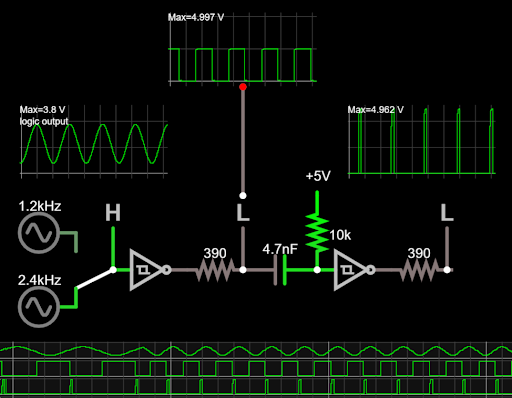square wave and pulse conversions (390Ω resistors model real schmitt outputs)

Next, we add a monostable oscillator. It outputs a fixed-width pulse each time it gets triggered.
We use the edge-pulses to trigger it. This effectively extends the length of the edge-pulses. If we trigger it quickly enough, the pulses will overlap, and cease to be pulses. The output will instead be constant.
The presence or absence of pulses is how we discriminate between frequencies. We can set the width (period) of the monostable output such that 1,200Hz will yield pulses, while 2,400Hz won't.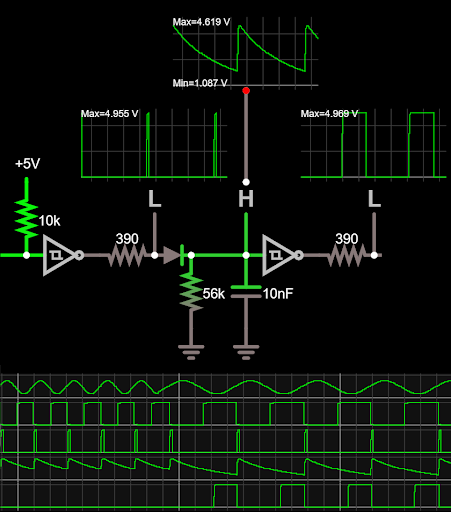monostable 1

This series of pulses isn't a very convenient signal to use for zero, so we'll convert it to a constant low signal instead.
We can use the pulses to trigger a second monostable. If the period of this monostable is long enough, we will again have overlapping pulses that give us a constant low output.

The period of this last monostable is somewhat critical as it impacts the maximum rate you can switch between high and low frequencies. The shorter the period, the faster the data can change. If it's too short though, there will be pulses in the zero signal. For this reason, we include a trim resistor that allows the period to be adjusted.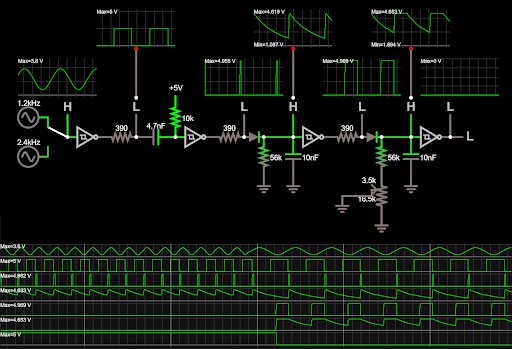link to simulation

Prior to this demodulation, we have a highpass, then a lowpassing amplifier. This brings the signal up to a level the schmitts can process, while also removing potential noise from outside the FSK range.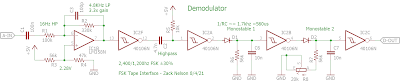our complete demodulator schematic

Combining the modulator and demodulator gives us the full modem.

## Saturday, October 16, 2021

### Storing data on a cassette using Arduino and Python (Differential Manchester encoding)

I've been building a retro computer, and it's gotten me interested in using cassettes as data storage. This poses an interesting challenge where binary information has to be converted into something that can be written to, and reliably read from, a cassette. We have to worry about immunity to noise (tape hiss), speed fluctuations (wow/flutter), and amplitude fluctuations (dropout).

Another limitation is frequency response. Our signal has to stay safely within the range of frequencies a tape can reproduce. This range can be as narrow as 400-4,000Hz for something like a microcassette. We could send a stream of bits at a safe 2kHz, but what if we then have a very long run of all zeros (or ones)? Our signal would dip below 400Hz, and our data would be lost.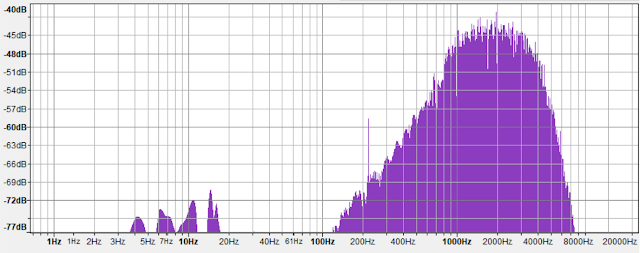frequency response of my Pearlcorder L400 microcassette recorder

One solution is to toggle our output at least once per bit. Two bits would give a full cycle and guarantee a minimum frequency of 1kHz. The presence of an additional toggle can represent a zero, and its absence a one. If every bit had an additional toggle, it would yield the maximum frequency of 2kHz. This is the basis of Differential Manchester encoding.Differential Manchester encoding - wikipedia

Besides it fitting nicely in a frequency range, Manchester has other advantages. Cassette recorders rarely concern themselves with the polarity of their signal (since it doesn't affect the sound) and will sometimes invert their output relative to their input. Manchester encoding only uses the presence of these "toggles" or edges, and is unaffected by being inverted.
Also, each bit spends an equal amount of time high and low. This means we have no DC offset. If the offset were irregular, our signal would drift up and down, making decoding more difficult.
It's fairly resilient in the face of speed warbles too, as we have an octave separating our ones and zeros. In other words, zero is always twice as fast as one. For comparison, one early modem standard used 1300Hz and 1700Hz for one and zero respectively.

So, Manchester encoding it is! This settles how we encode individual bits, but not how we structure our data. I chose to mimic the standard serial packet structure of "8n1". This means a zero starting bit, 8 data bits, no parity bit, and a one stop bit. This makes it easy to figure out exactly how the data is aligned when receiving.
I opted to add a calibration tone to the beginning of my files. This gives the receiver time to detect the amplitude, and more importantly, the frequency of the signal. This tone is simply a long string of ones. The starting bit (zero) of the first byte signifies the end of the tone.

I've written a python script that will take a binary file and output a Manchester encoded audio file that can be recorded directly onto a cassette.

Python encoder:
``````#Converts binary file to Differential Manchester encoded audio
# outputs 32kHz, 8bit, mono WAV. 8N1 format at 3200 baud
# includes calibration tone, and checksum. Zack Nelson 2021
import struct, os
from sys import argv

smplrate = 32000 #Hz
baud = 3200 #needs integer ratio between baud and sample rate

#functions-------------------------------------------------
#each bit starts by inverting the output
#zeros will invert again in the middle
def out_bit(bit):
global bit_status
bit_status = not bit_status #toggle
for x in range(2): #2 half-cycles
for y in range(int(smplrate/baud/2)): #samples
if bit_status: buf.append(0xD8) # hi
else: buf.append(0x28) # lo
#toggle if bit 0
if x == 0 and not bit: bit_status = not bit_status

def out_byte(byte):
out_bit(0) #start bit
for i in range(8):
out_bit(bool(byte & (1<<7)))
byte <<= 1
out_bit(1) #stop bit
#---------------------------------------------------------

except IndexError:
print("Input file needed")
exit(2)

fi = open(argv,'rb') #open input
fo = open(os.path.splitext(argv)+".wav", 'wb+') #open output

fi.close()
buf = []

bit_status = False
checksum = 0

for i in range(smplrate): buf.append(0x80) #silence
for i in range(256): out_bit(1) #calibration bits

for byte in file: #add all bytes
checksum += byte
out_byte(byte)

for i in range(smplrate): buf.append(0x80)#silence

fo.write(str.encode("RIFF"))
fo.write((len(buf) + 36).to_bytes(4, byteorder='little')) #length in bytes
fo.write(str.encode("WAVEfmt "))
fo.write((16).to_bytes(4, byteorder='little')) #Length of format data
fo.write((1).to_bytes(2, byteorder='little')) #PCM
fo.write((1).to_bytes(2, byteorder='little')) #Number of chans
fo.write((smplrate).to_bytes(4, byteorder='little')) #Sample Rate
fo.write((smplrate).to_bytes(4, byteorder='little')) #Sample Rate * bits * chans / 8
fo.write((1).to_bytes(2, byteorder='little')) #8bit mono
fo.write((8).to_bytes(2, byteorder='little')) #Bits per sample
fo.write(str.encode("data"))
fo.write(len(buf).to_bytes(4, byteorder='little')) #length in bytes

fo.write(struct.pack('B'*len(buf), *buf)) #write audio to file
fo.close()
``````

## Hardware Interface

Now that we can store data onto a tape, we need a way to read it back. First we'll focus on the hardware required to connect the cassette recorder to a computer.

To read from a tape, the audio is first highpassed. This reduces potential DC offset, and noise from motor rumble. The audio is then amplified, bringing the tape's ~1V line-level output closer to the 5V we want for the digital signal. The amplification stage also lowpasses the audio, reducing some hiss and noise outside of the range of our signal.
Next the audio is passed through a schmitt trigger. This transforms the smooth audio to a rigid, digital signal by comparing it to two thresholds. If the audio signal goes above the high (2.6V) threshold, the output is a digital one. If it goes below the low threshold (1.5V), the output is a zero. If the signal hangs out between the two, the output does not change. This provides some noise immunity. As long as the noise doesn't swing enough to push the signal over the wrong threshold, it will simply be ignored.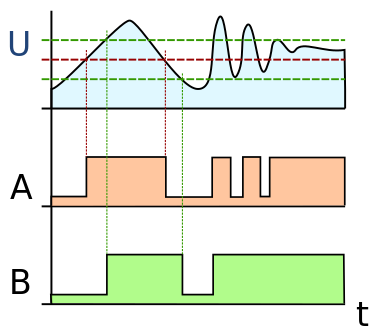Schmitt trigger input(U) and thresholds (A, B) - wikipedia

Now we have a digital signal, but it's still Manchester encoded. I selected an Arduino to run a proof-of-concept decoding program. It takes in the digital signal from our interface board (via pin D2) and outputs the decoded bytes over serial.
To do this, it listens to part of the calibration tone, and calculates the signal's timing. It uses this timing to discern ones from zeros. Three edges close together count as a zero. Two edges far apart count as a one.
When it detects the first zero (start bit), it begins constructing and transmitting bytes.
It has the ability to detect and report framing errors (incorrect start/stop bit placement), and invalid edge patterns. It's unable to recover from these errors though. It would be possible to correct framing issues by buffering bits and searching for valid frames within the buffer.

Arduino Decoder:
``````// Differential Manchester decoder
// Zack Nelson
const byte pulsePin = 2; //interrupt input

int byte_count = 0; //count for printing newlines
uint32_t last_ts = 0; //timestamp of prev edge
byte edge_count = 0; //edges per bit
byte bit_count = 0;
byte rec_Byte = 0;

//calibration-----------------------------------
unsigned int hi_threshold = 0; //hi pulse in uS
unsigned int cal_count = 0;
unsigned int cal_ts = 0;

void setup() {
pinMode(pulsePin, INPUT);

attachInterrupt(digitalPinToInterrupt(pulsePin), count, CHANGE );

Serial.begin(230400);
Serial.print("Start. ");

while(!hi_threshold); //Calibration done--------------------------
Serial.print("High threshold(us): ");
Serial.println(hi_threshold);
}

void loop() { }

void count() { //gets called on every transition of data pin
if (!hi_threshold){ //Calibration---------------------------------
if (cal_count == 32) cal_ts = 0; //skip 0-31 readings
cal_ts += (micros() - last_ts); //average 16 pulses
if (++cal_count == 48) hi_threshold = cal_ts / 21; //calc 75%
bool bit_val = ((micros() - last_ts) > hi_threshold); //hi or lo?

if (++edge_count > 2) { //error
Serial.println("Edge cnt err");
edge_count = 1;
}

//low bit = 2 fast pulses, high = 1 slow pulse
if ((!bit_val && edge_count == 2) || (bit_val && edge_count == 1)){
edge_count = 0;
}
}

last_ts = micros();
}

void bitDone(bool bit_val) {
//start bit lo, 8 bits MSB first, stop bit hi
if (bit_val) rec_Byte |= (0x80 >> (bit_count-1));

if (bit_count == 0 && bit_val) Serial.println("Start err");
else if (bit_count == 9 && !bit_val) Serial.println("Stop err");

if (++bit_count == 10) { //complete byte?
//Uncomment to print hex
/*if (rec_Byte < 16) Serial.print(0); //leading zero
Serial.print(rec_Byte, HEX);
Serial.print(", ");
if (++byte_count % 16 == 0) Serial.println(""); */
Serial.print((char)rec_Byte); //print ASCII character

bit_count = 0;
rec_Byte = 0;
}
}``````

Files are available on my github page.

Here are some images of my setup to read from a microcassette. I was able to use it to read data out at around 3000 baud.

## Sunday, June 21, 2020

### DIY NES to Atari Controller Adapter

I wanted to use my favorite NES controller with my controller-less Atari 65XE and 130XE. The NES controller uses a shift register, while the Atari expects 5 simple buttons. I wrote a simple Arduino program to convert one to the other. Using an Arduino mini, it fits in this little inline enclosure.

```#define strobe 3 //PORTD#define clk 4#define data 2
void setup() {  DDRD = 1 | (1<<strobe) | (1<<clk); // NES outputs}
void pulse_pin(byte pin, byte del) {  PORTD |= (1<<pin); //set  delayMicroseconds(del);  PORTD &= ~(1<<pin); //clear  delayMicroseconds(del);}
void loop() {  byte btns = 0;  pulse_pin(strobe, 10); //latch the controller
for(int i = 0; i < 8; i++) { //read 8 bits    btns = btns << 1 | !(PIND & (1<<data));    pulse_pin(clk, 10); //clock next bit  }
// atari output d12-d8, open collector  DDRB = (btns & 0xf) | (!!(btns & 0xc0) << 4); // dpad + A||B}```

## Thursday, June 11, 2020

### DIY 1702a Programmer

I've resumed working on the PAiA 8700 computer. It uses some very old, and hard to program EPROMs: 1702As. They require +37V, +47V, +59V and ground to program.

I've come up with a relatively easy to build DIY programmer. It doesn't require a parallel port, or dozens of transistors. It's Arduino based, and uses mostly common parts.
Here's what it looks like:And here's a schematic:A boost converter daughter board is used to generate a high voltage, over 60V. It then gets regulated down to the various voltages needed. IC1 (TL783) has to be calibrated to +47V, then the other voltages will fall into place.
An Arduino nano is able to manipulate the high voltages through three ULN2003 transistor arrays, and one IRF520 MOSFET.

Right now the code is very crude, but functional. It's on pastebin here. The binary for the 1702A is included in the Arduino program. On power up/reset the programming sequence begins. It's finished when the pin 13 led stops flashing.

### Pollard Syndrum Schematic Redraw

The original Syndrum schematic isn't the most legible in terms of quality or layout.
I redrew it so that it made more sense to me. Here it is:## Friday, May 22, 2020

### Boss DR-110 Clap Pulse Generator (trigger adapter)

Here's a circuit I came up with a while back. I had a DR-110 with a dead digital board. The digital board is responsible for generating all the triggers in the system, and it generates some very specific triggers for the clap circuit.

CPI is three 1ms long pulses, every 10ms (100hz).
CPII is a separate pulse also 1ms long, that falls 10ms later.

I re-implemented this in the following circuit.
Traces from top to bottom are CPI, CPII, and Trigger in.

The traces are from a simulation of the circuit, that you can play with in real time here.

Breaking down the circuit, we start at C8 and R11. These form a hipass filter for the incoming trigger. This ensures that long triggers will be handled the same way as short triggers.

IC6A & B are schmitt NAND gates and they form an SR latch. It is set by an incoming trigger, and is reset by the CPII pulse. The output is used to enable the generation of the CPI signal.

IC6C is the core of an oscillator that can be enable/disabled from pin 8. It works by charging and discharging C9.
R14 sets the charging rate of C9, and determines the low time between pulses.
R15 and D4 set the discharge rate, and determine the length of the pulses.
R12 & R13 help bias C9 near the threshold voltage of IC6C. This ensures that the first pulse will be close in length to the following pulses. If C8 had to charge all the way up from ground, the first pulse would be much longer.

IC7 is a counter, and is keeping track of how many pulses have been seen on CPI.
Once the end of the third pulse is counted, output QC goes high. This resets the SR latch, disabling the oscillator, and very quickly stopping the fourth trigger.
Output QC is also used as our CPII signal. To limit it to 1ms in length, we use it to charge C7 through R9. After about 1ms, C7 has charged enough to trigger the CLR pin of the counter. This causes output QC to go low again and discharge C7.

So, with some simple tweaking, this circuit can generate different lengths, rates, and numbers of pulses.

## Thursday, May 7, 2020

### Korg Monotron Delay PCB Component Labels

Even though it's old-hat, I'd like to try modifying the Korg Monotron Delay.
Korg was nice enough to share their schematic, but not the board layout. Also, the board itself isn't labeled.
I need to know what I'm working with, so I used a multimeter, and some educated guesses to draw the following. I labeled the rough sections of the circuit, so that it's a little easier to find what you're looking for. Be warned that it's not perfect, but should be a decent starting point.